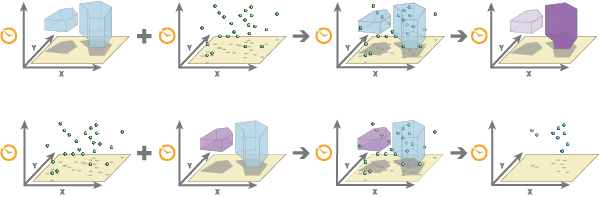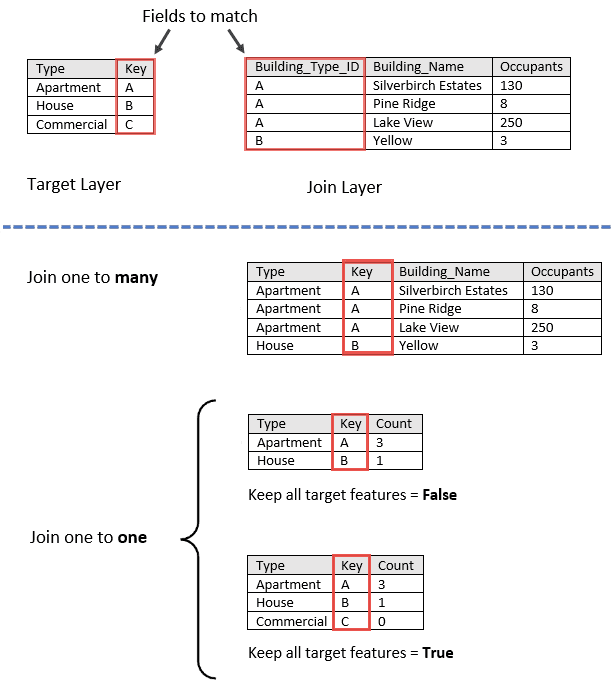# Spatiotemporal join

Spatiotemporal Join transfers fields from one DataFrame to another based on spatial, temporal, and field attribute relationships, or some combination of the three. Optionally, statistics can be calculated for the joined records.## Usage notes

• A spatial join matches records from the input DataFrames (`target_dataframe` and `join_dataframe`) based on their spatial relationships. A temporal join matches records from the target DataFrames based on their temporal relationships. An attribute join matches records based on field values.

• You can join records based on a spatial relationship, a temporal relationship, an attribute relationship, or a combination of the three.

OptionDescription
`setSpatialRelationship()`The spatial relationship that will determine whether records are joined to each other. The available relationships depend on the geometry type (point, linestring, or polygon) of the DataFrames being joined. Available spatial relationships are as follows:
• Intersects
• Equals
• NearPlanar
• NearGeodesic
• Contains
• Within
• Touches
• Crosses
• Overlaps
`setTemporalRelationship()`The temporal relationship that will determine whether records are joined to each other. The available relationships depend on the time type (instant or interval) of the DataFrames being joined. Available temporal relationships are as follows:
• Meets
• MetBy
• Overlaps
• OverlappedBy
• During
• Contains
• Equals
• Finishes
• FinishedBy
• Starts
• StartedBy
• Intersects
• Near
• NearBefore
• NearAfter
`setAttributeRelationship()`The attribute relationship that will determine whether records are joined to each other. Records are matched when the field values in the join DataFrame are equal to the field values in the target DataFrame.
• If the target DataFrame and join DataFrame are in different coordinate systems, the coordinate system of the target DataFrame will be used.

• When `'NearPlanar'` is specified with `setSpatialRelationship()`, it is required that the target DataFrame's geometry has a projected coordinate system or the tool will fail. You can transform your data to a projected coordinate system by using ST_Transform.

• If multiple join records match the same target record, you can decide whether all the matching records will be joined (`setJoinOneToMany()`) or all the matching records will be summarized together (`setJoinOneToOne()`) as follows:

• One-to-many—Joins all the matching records in the join DataFrame to the target DataFrame. The resulting DataFrame will contain multiple records of the target record. If `True` is specified for `setLeftJoin()`, all input records are written to the output DataFrame.

• One-to-one—Summarizes all of the matching join records with each record in the target DataFrame. Only the records that have a match will be included in the summary and output. The count of joined records will be added, in addition to other standard statistics such as sum, minimum, maximum, range, mean, variance, standard deviation, first, and last, as well as string statistics including count, any, first, and last. By default, only those join records that have the specified relationships will be maintained in the output DataFrame (inner join). If `True` is specified for `setLeftJoin()`, all input records are written to the output DataFrame.

• To add first or last statistic field in the output, time needs to be enabled on the input DataFrames.

• Examples of a one-to-many and one-to-one attribute join are shown below. In this example, the one-to-one join only includes the count; additional statistics that can be calculated using `addSummaryField()`.• You can optionally build an expression to join records using `setJoinCondition()`. This should always be used with a spatial, temporal, or field attribute relationship, or some combination of the three. If you specify an expression, only records that meet the condition will be used. For example, using the expression `\$target['Magnitude'] > \$join['Explosion']`, you only join target records if the `Magnitude` field is greater than the `Explosion` field in the join record. See Arcade expressions for more information.

• You can optionally return the the spatial distance and temporal difference of the joined records by setting the `includeDistance()` parameter. Spatial distance calculations are returned in a field named `distance`, a double column. The distance method used is determined by the `spatial_relationship` parameter. For example, if you specify `'NearGeodesic'`, the geodesic distance method is applied when calculating the distance. Similarly, temporal difference calculations are returned in a field named `timegap`, represented as milliseconds in a long column. The output fields and values are outlined below:

• The `distance` field can have the following values:

• 0—This represents the target and join geometries intersect
• Greater than 0—This represents the distance between the join and target records geometries. The default distance unit is meters.
• Null values—This represents the case where geometries are further apart than the `near_distance` parameter in the `setSpatialRelationship()` setter.
• The `time_gap` field can have the following values:

• Less than 0—This represents the time difference between the target and join records. Negative values mean the join record occurs before the target record.
• 0—This represents times that intersect.
• Greater than 0—This represents the time difference between the target and join records. Positive values mean the target records occurs before the join record.
• Null values—This represents the case where records did not mean the join condition. For example, they are further apart than the `near_duration` in the `setTemporalRelationship()` setter, or the ordering of the target and join record did not match given relationship, such as `NearBefore`.

## Results

The fields in the output DataFrame differ depending on the join operation.

### One-to-many join

• The following fields are included in the output DataFrame when running a one-to-many join:

• All fields from the target DataFrame
• All fields from the joined DataFrame

If the same non-geometry field name exists in the target and join DataFrame, the joined field will start with the word join (for example join_fieldname).

If the same geometry field name exists in the target and join DataFrame, the result will have multiple geometry fields with the same name, where the first geometry field in the result schema is from the target DataFrame and the second geometry field is from the joined DataFrame. You can rename the geometry fields of your input DataFrames to be unique before joining to avoid confusion.

If you set the `includeDistance()` parameter, your output will have fields named `distance` and `time_gap` representing the spatial distance and temporal difference between the joined features.

### One-to-one join

In addition to all fields from the target DataFrame, the following fields are included in the output records when running a one-to-one join:

FieldDescription
`count`The count of records that have been joined.
`<statistic>_<fieldname>`Specified statistics will each create an attribute field, named in the following format: statistic_fieldname. For example, the maximum and standard deviation of the id field is MAX_id and SD_id.

## Performance notes

Improve the performance of Spatiotemporal Join by doing one or more of the following:

• Only analyze the records in your area of interest. You can pick the records of interest by using one of the following SQL functions:

• ST_Intersection—Clip to an area of interest represented by a polygon. This will modify your input records.
• ST_EnvIntersects—Select records that intersect an envelope.
• ST_Intersects—Select records that intersect another dataset or area of intersect represented by a polygon.
• Make the join conditions as selective as possible. For example, a near join of 1 kilometer will perform better than a near join of 5 kilometers on the same DataFrame.
• Add more than one join condition. Additional join conditions make the join more selective. For example, a near join of 1 kilometer and 1 hour will perform better than a near join of 1 kilometer on the same data.
• Apply a join expression.
• Calculating the `distance` and `time_gap` fields may increase processing time.
• Planar distance calculation (specified with the `'NearPlanar'` option in `setSpatialRelationship`) will perform better than geodesic.
• If the data is in geographic coordinate system but for small, local areas, project it to a local coordinate system, and specify `'NearPlanar'` in the `spatial_relationship` parameter. You can transform your data to a projected coordinate system by using ST_Transform.

## Syntax

For more details, go to the GeoAnalytics Engine API reference for spatiotemporal join.

SetterDescriptionRequired
`run(target_dataframe, join_dataframe)`Runs the Spatiotemporal Join tool using the provided DataFrames.Yes
`setAttributeRelationship(attribute_relationship)`Sets a target field, relationship, and join field used to join equal attributes.One of `setAttributeRelationship()`, `setSpatialRelationship()`, or`setTemporalRelationship()` is required.
`setJoinOneToMany()`Sets the join operation to one to many. If multiple join records are found that have the same relationships with a single target record, the fields associated with the multiple join records will be aggregated using the specified summary statistics.One of `setJoinOneToOne()` or`setJoinOneToMany()` are required.
`setJoinOneToOne()`Sets the join operation to one to one. If multiple join records are found that have the same relationships with a single target record, there will be multiple copies (records) of the target record joined to each matching join record.One of `setJoinOneToOne()` or`setJoinOneToMany()` are required.
`setSpatialRelationship(spatial_relationship, near_distance=None, near_distance_unit=None)`Sets the spatial relationship used to spatially join records.One of `setAttributeRelationship()`, `setSpatialRelationship()`, or `setTemporalRelationship()` is required.
`setTemporalRelationship(temporal_relationship, near_duration=None, near_duration_unit=None)`Sets the temporal relationship used to temporally join records.One of `setAttributeRelationship()`, `setSpatialRelationship()`, or `setTemporalRelationship()` is required.
`addSummaryField(summary_field, statistic, alias=None)`Adds a summary statistic of a field in the join DataFrame to the result DataFrame.No
`setJoinCondition(join_condition)`Sets a condition to specified fields using an Arcade expression. Only records with fields that meet this conditions will be joined.No
`setLeftJoin(left_join=True)`Specifies whether all target records will be returned in the result DataFrame (known as a left outer join) or only those that have the specified relationships with the join records (inner join). Left outer join can be used with one-to-one join or for one-to-many join.No
`includeDistance(include=True, distance_unit="Meters")`Include spatial distance and/or temporal difference calculated between target and join features. Set `include` to `True` to include `distance` and/or `timegap` fields in the result. The `distance_unit` can be chosen from `'Feet'`, `'Kilometers'`, `'Meters'`, `'Miles'`, `'NauticalMiles'`, or `'Yards'`.No

## Examples

### Run Spatiotemporal Join

Python
Use dark colors for code blocksCopy
``````# Log in
import geoanalytics

# Imports
from geoanalytics.tools import ReconstructTracks
from geoanalytics.tools import SpatiotemporalJoin
from geoanalytics.sql import functions as ST

# Path to the hurricane track points data and USA counties data
hurricanes_data_path = r"https://services2.arcgis.com/FiaPA4ga0iQKduv3/arcgis/rest/" \
"services/IBTrACS_ALL_list_v04r00_points_1/FeatureServer/0"
counties_data_path = "https://services.arcgis.com/P3ePLMYs2RVChkJx/ArcGIS/rest/services/"\
"USA_Counties_Generalized/FeatureServer/0"

# Create DataFrames for hurricane track points and USA counties
hurricanes_df = hurricanes_df.withColumn("usa",
ST.env_intersects("shape",
xmin=-14165303, xmax=-7384568,
ymin=1947768, ymax=7426938)).where("usa=true")

# Reconstruct hurricane points into tracks using Reconstruct Tracks
rt_result = ReconstructTracks() \
.setTrackFields("NAME") \
.setDistanceMethod(distance_method="Geodesic") \
.run(dataframe=hurricanes_df)

# Use Spatiotemporal Join to join the hurricane tracks to each county
join_result = SpatiotemporalJoin() \
.setLeftJoin(left_join=True) \
.setJoinOneToOne()\
.setSpatialRelationship(spatial_relationship="Intersects") \
.run(target_dataframe=counties_df, join_dataframe=rt_result)
``````

### Plot results

Python
Use dark colors for code blocksCopy
``````# Plot the join result and visualize the frequency of hurricanes for each county
result_plot = join_result.st.plot(cmap_values="COUNT",
cmap="coolwarm",
legend=True,
figsize=(14,8),
geometry="shape",
basemap="light")
result_plot.set_title("USA counties visualized by count of intersecting hurricanes")
result_plot.set_xlabel("X (Meters)")
result_plot.set_ylabel("Y (Meters)")
result_plot.set_xlim(left=-14200000, right=-7300000)
result_plot.set_ylim(bottom=2100000, top=7200000);``````

## Version table

ReleaseNotes

1.0.0

Tool introduced

1.1.0

Added support for left one-to-many join.

1.2.0

Added support for spatial distance and temporal difference in near join.